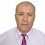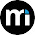Ab initio Calculations Using Wien2k Code

### Last Posts

10/recent/ticker-posts

# Calculating the cohesive and formation energies (according to an article)

The procedure to calculate the cohesive and formation energies is taken from the following article:

http://sci-hub.tw/http://www.sciencedirect.com/science/article/pii/S2211379712000137

The author has calculated the cohesive and formation energies for the compound BaM2 (M=Rh, Pd and Pt)

The formation energy:

The energy of formation per atom is defined as:

where x and y are the numbers of Ba and (Rh, Pd, Pt) atoms in unit cell, respectively. EBaM2 total ; EBa solid and EM solid are the calculated total energies of the C15-type BaM2 compounds, Ba, and M, respectively. During calculation, Ba and M are taken as body-centered cubic structure (space group Im-3m) and cubic close-packed (space group Fm-3m), respectively.

Analyzing the results:

The calculation of the formation energy gives the following values 0.107 eV/atom, 0.660 eV/atom and 0.823 eV/atom for BaRh2, BaPd2 and BaPt2, respectively, which are all negative. Such a negative value of Efor implies that the BaM2 phases are energetically favorable from the point of view of thermodynamics, and this stable structure is the most likely to be formed during experiments.

The cohesive energy:

The cohesive energy is a measure of the strength of the forces that bind atoms together in the solid state and is descriptive in studying the phase stability. The cohesive energy EBaM2 coh of BaM2 is defined as the total energy of the constituent atoms minus the total energy of the compound:

where Ecoh is the total energy of the unit cell used in the present calculation, x and y are the numbers of Ba and (Rh, Pd, Pt) atoms in unit cell, respectively. EBaM2 total refers to the total energy of C15-type BaM2 in the equilibrium configuration and EBa atom; EM atom are the isolated atomic energies of the pure constituents. The computed cohesive energies are 6.101 eV/atom, 4.774 eV/ atom and 6.512 eV/atom for BaRh2, BaPd2 and BaPt2, respectively.

1.Thanks for sharing this quality information with us. I really enjoyed reading. Will surely going to share this URL with my friends. documenti per iseeu

2.Really impressed! Everything is very open and very clear clarification of issues. It contains truly facts. Your website is very valuable. Thanks for sharing. formation anglais dif lyon

1.3.where can we find this values ??? i mean Etot and partial Eatom

4.In cohesive energy the positive value means stable???

5.Useful information. Check out our Vehicle Tracking